# Price Determination

Courses Info

Level: AS Levels, A Level, GCSE – Exam Boards: Edexcel, AQA, OCR, WJEC, IB, Eduqas – Economics Revision Notes

### Equilibrium

The interaction of supply and demand determine the equilibrium price and quantity. This would not change unless there is a change in the conditions of demand / supply.

Equilibrium occurs when the quantity demanded equals the quantity supplied.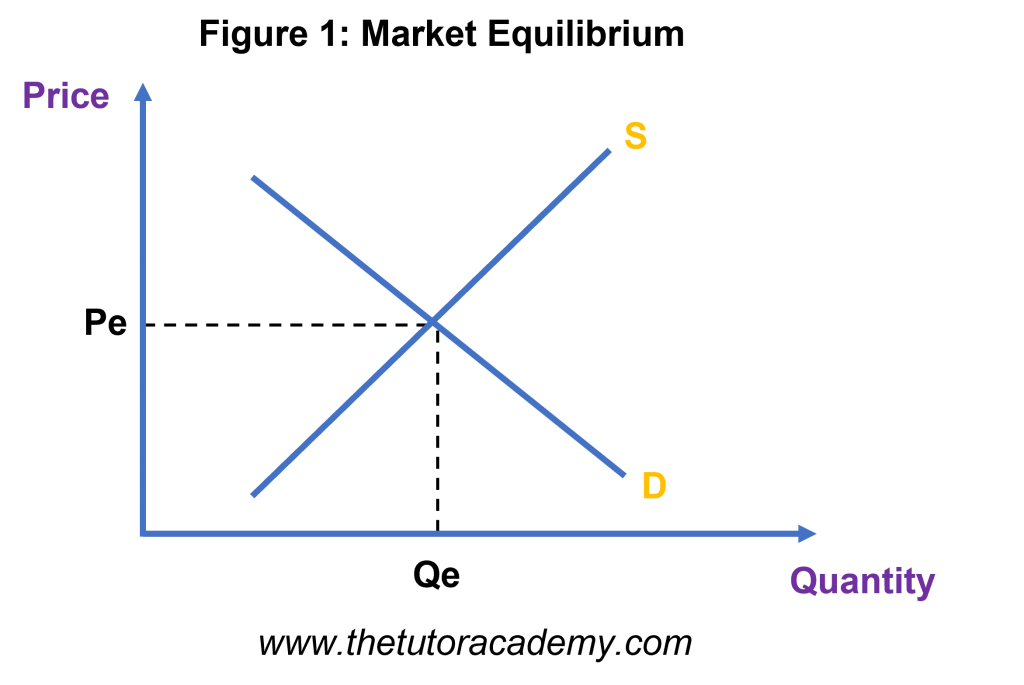### Excess Demand

This occurs when the quantity demanded outweighs the quantity supplied at the existing price.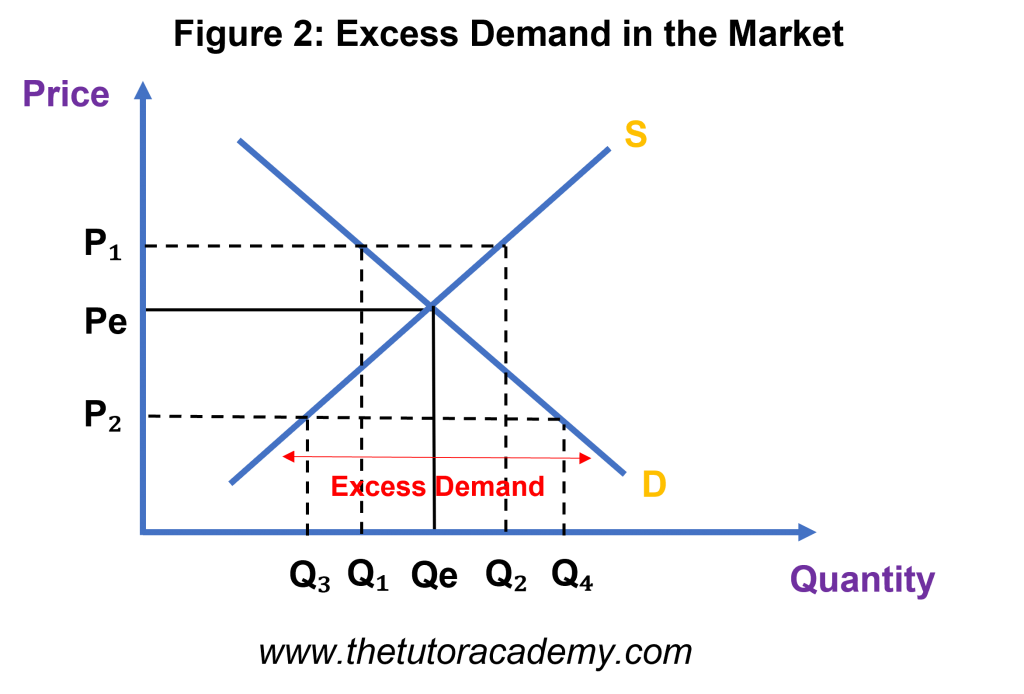Excess demand will occur if the price is below the equilibrium price of Pe. In order to eliminate the excess demand, market forces will cause prices to rise which will lead to an extension of supply and a contraction in demand.

### Excess Supply

This occurs when the quantity supplied outweighs the quantity demanded at the existing price.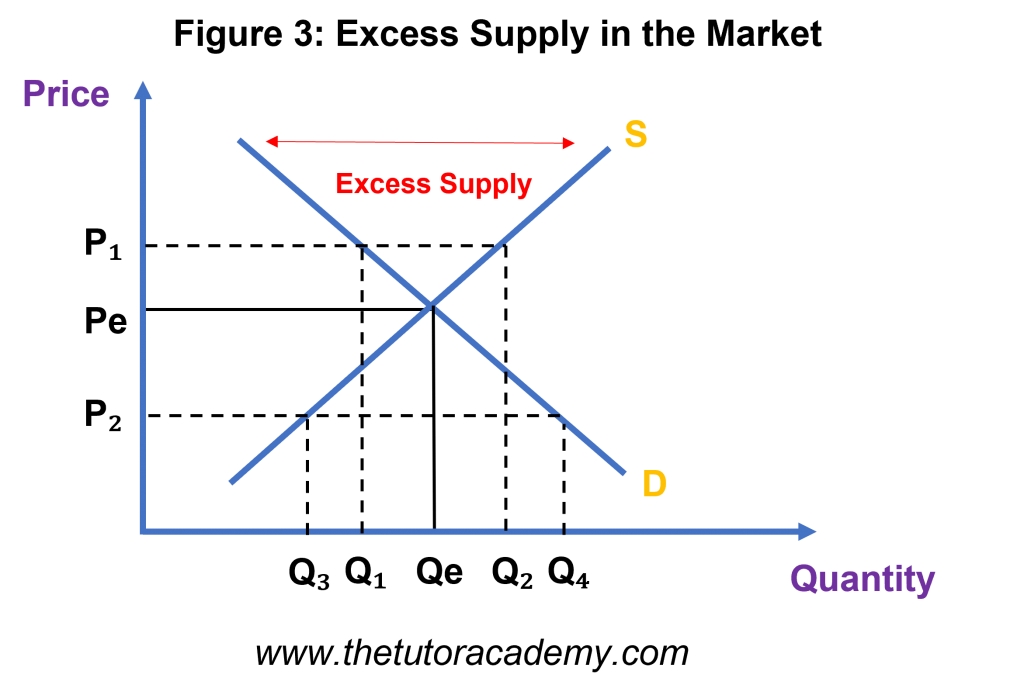Excess supply will occur if the price is above the price of the equilibrium price Pe. In order to eliminate excess supply, market forces will cause prices to fall which will lead to an extension of demand and a contraction in supply

### Changes in Equilibrium Price caused by changes in Demand

Increase in Demand – an increase in demand will result in a larger amount of quantity, a rise in price and a rightward shift in the demand curve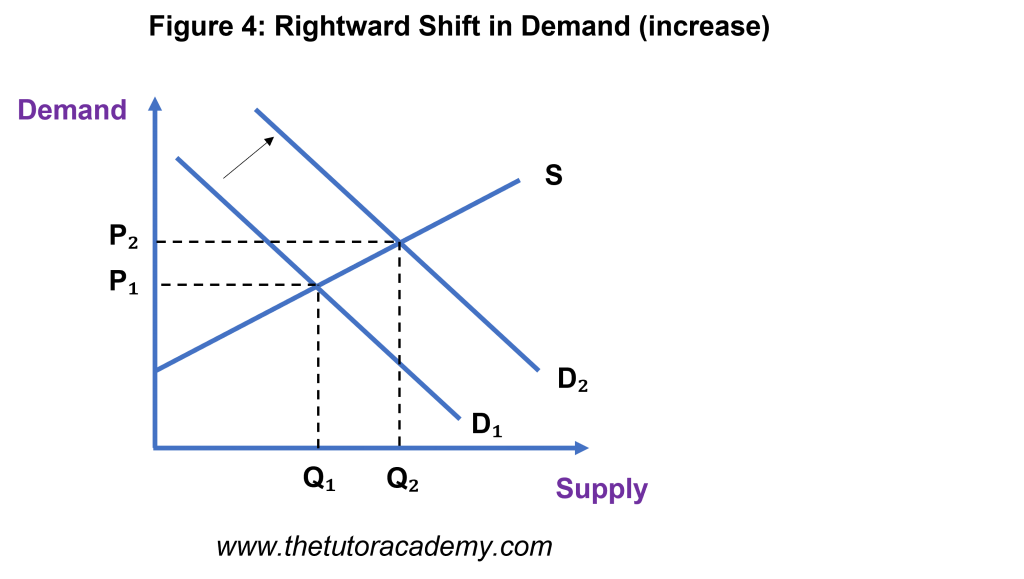Decrease in Demand
– a decrease in demand will result in a smaller amount of quantity, a fall in prices and a leftward shift in the demand curve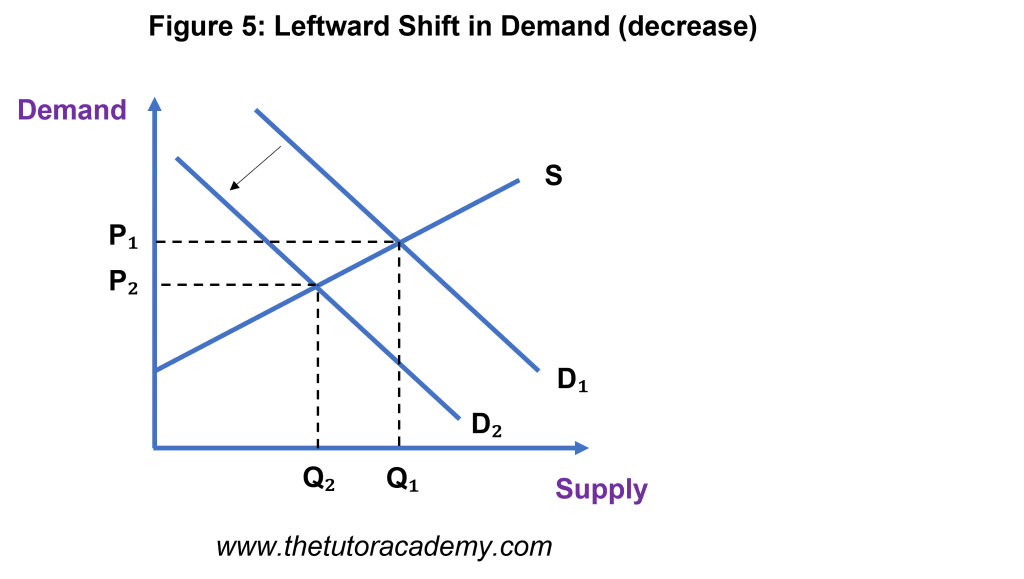### Changes in Equilibrium Price caused by changes in Supply

Increase in Supply – an increase in supply will cause the quantity to rise, a fall in price and a rightward shift in the supply curve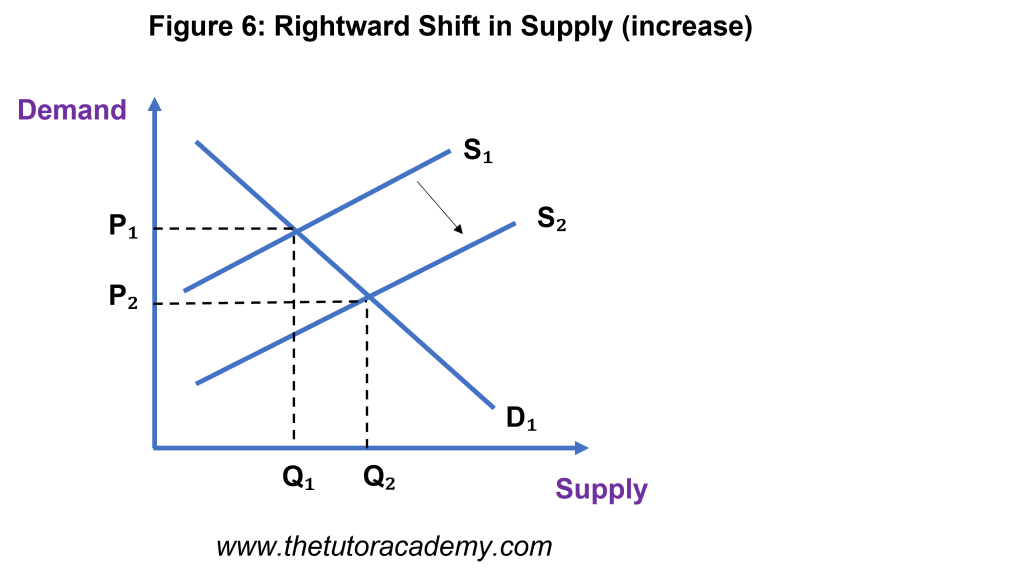Decrease in Supply – a decrease in supply will cause the quantity to fall, a rise in price and a leftward shift in the supply curve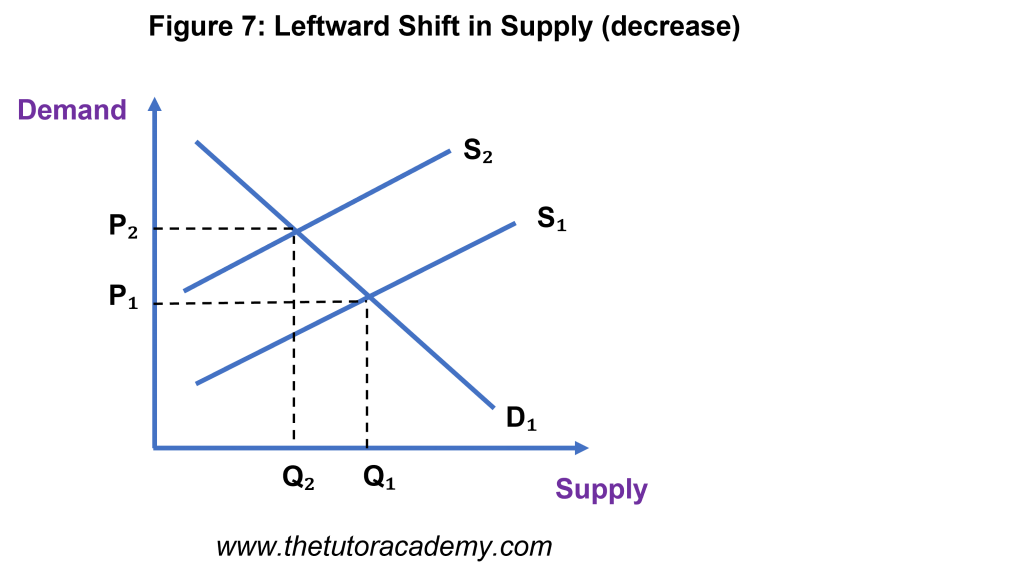Quick Fire Quiz – Knowledge Check

1. Using a diagram, explain what is meant be ‘Equilibrium’ (4 marks)

2. Using a diagram, explain how excess demand would be occur (4 marks)

3. Using a diagram, explain how excess supply would be occur (4 marks)

4. Explain how changes in the demand would impact the equilibrium price (4 marks)

5. Explain how changes in the supply would impact the equilibrium price (4 marks)

Next Revision Topics

A Level Economics Past PapersEconomics Tutor